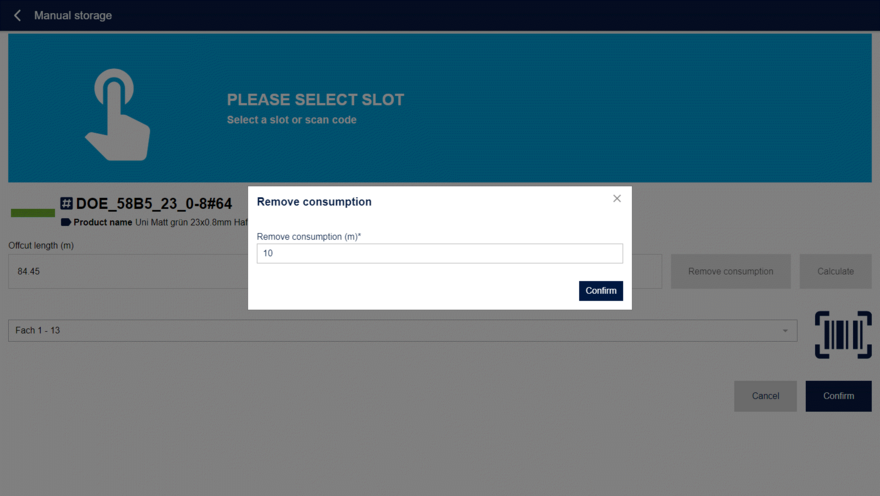# Determination of the remaining length

The remaining length (1) known to the materialAssist is displayed in the manual storage dialog.

If the remaining length has changed and is known, it can be registered directly.

Otherwise, the consumption can be subtracted by clicking on subtract consumption(2) or the length can be calculated by measuring the inner and outer diameter by clicking on calculate(3).

## Subtract consumption

After clicking on the subtract consumption (2) button, a dialog opens in where the current consumption can be filled in. This value can be read in the user interface of many edge banding machines.

The value is subtracted from the currently known remaining length.Determination of the remaining length by subtracting consumption

## Measurement of the inner and outer diameter

After clicking on the button calculate(3) a dialog opens in which the measured inner and outer diameter can be registered.

Note: This function is very useful for the initial setup of the edgeband storage.

materialAssist calculates the residual length from the two entered values and the stored edge thickness and saves it automatically.